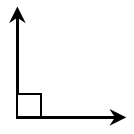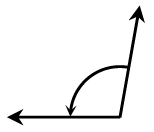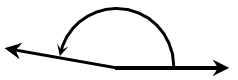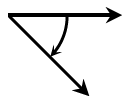### Home > GB8I > Chapter 1 Unit 1 > Lesson INT1: 1.1.3 > Problem1-31

1-31.Estimate the size of each angle below to the nearest $10º$. A right angle is shown for reference so you should not need a protractor. Then classify each angle. Read the Math Notes box in this lesson if you need a reminder about angle classification.

1.1.1.$\approx100º$
obtuse angle

$\approx170º$
obtuse angle

$\approx45º$
acute angle